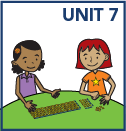top of page

• Use addition, subtraction, multiplication, and division to solve story problems

• Solve multiplication and division facts accurately and efficiently

• Multiply 1-digit numbers by multiples of 10, e.g., 3 x 70

• Add and subtract 2- and 3-digit numbers

• Compare, recognize, and generate equivalent fractions and place them on a number line

• Estimate and measure in time, liquid volume, and masses of objects

• Round numbers to nearest 10 or 100

• Tell time to the minute

• Estimate and measure liquid volume and mass in metric units

• Solve area and perimeter problems

• Identify and construct different kinds of quadrilaterals

• Sort and classify shapesbottom of page